# Electric Potential Energy and Electric Potential Physics 231

• Slides: 35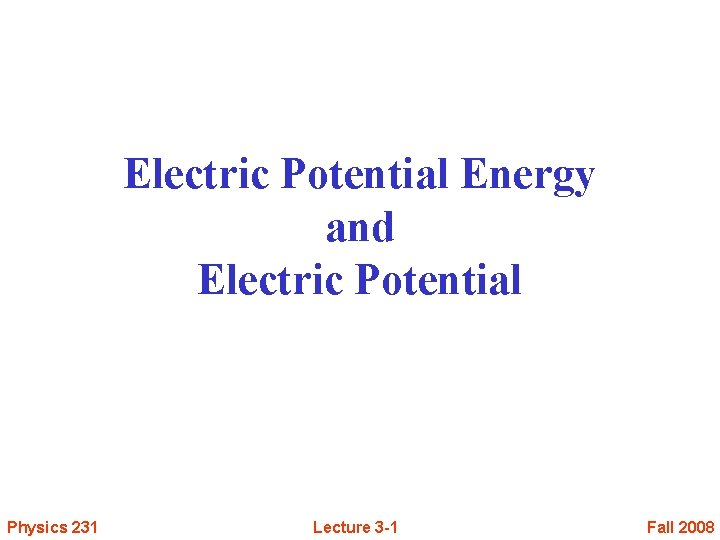Electric Potential Energy and Electric Potential Physics 231 Lecture 3 -1 Fall 2008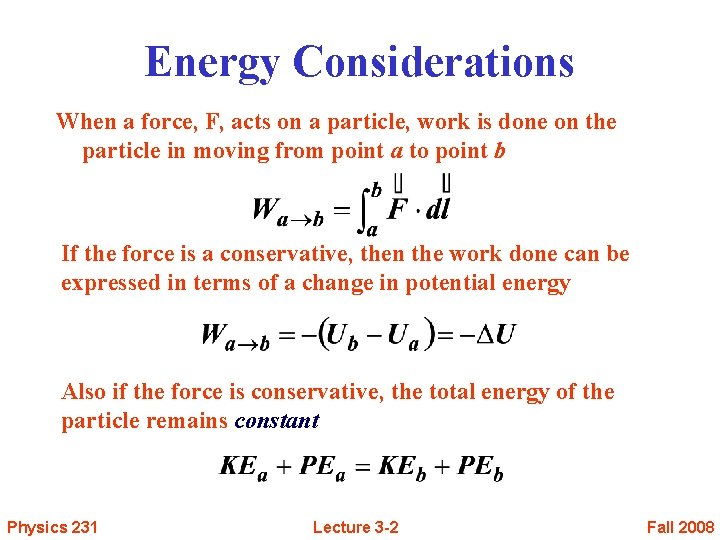Energy Considerations When a force, F, acts on a particle, work is done on the particle in moving from point a to point b If the force is a conservative, then the work done can be expressed in terms of a change in potential energy Also if the force is conservative, the total energy of the particle remains constant Physics 231 Lecture 3 -2 Fall 2008Work Done by Uniform Electric Field Force on charge is Work is done on the charge by field The work done is independent of path taken from point a to point b because The Electric Force is a conservative force Physics 231 Lecture 3 -3 Fall 2008Electric Potential Energy The work done by the force is the same as the change in the particle’s potential energy The work done only depends upon the change in position Physics 231 Lecture 3 -4 Fall 2008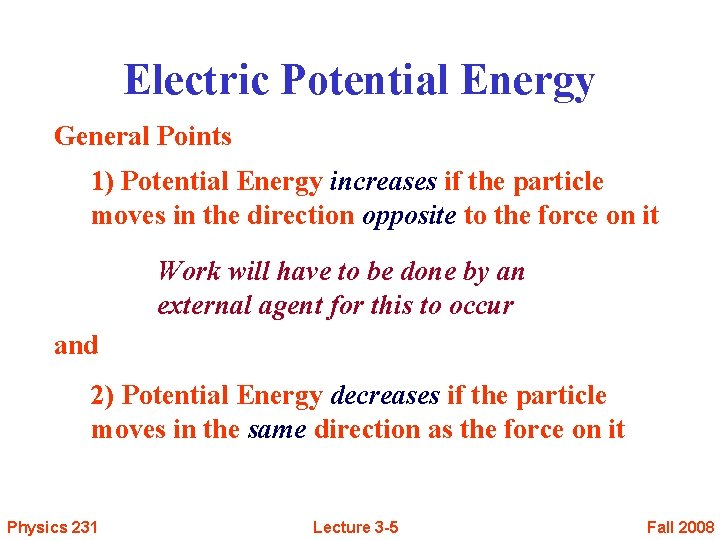Electric Potential Energy General Points 1) Potential Energy increases if the particle moves in the direction opposite to the force on it Work will have to be done by an external agent for this to occur and 2) Potential Energy decreases if the particle moves in the same direction as the force on it Physics 231 Lecture 3 -5 Fall 2008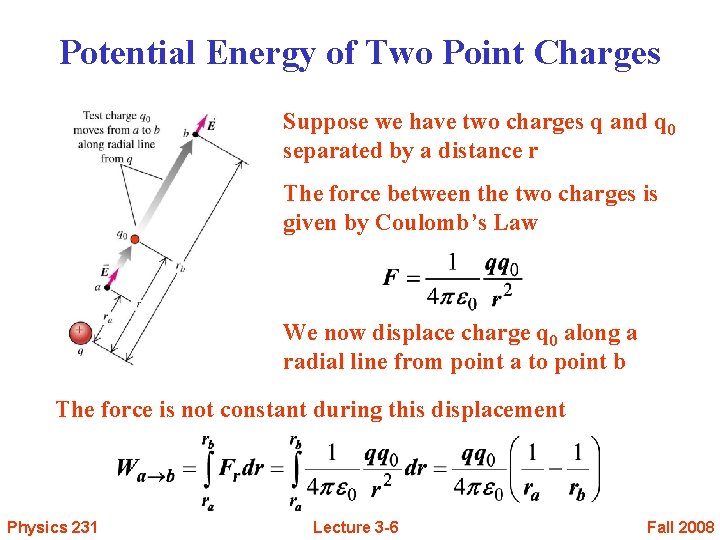Potential Energy of Two Point Charges Suppose we have two charges q and q 0 separated by a distance r The force between the two charges is given by Coulomb’s Law We now displace charge q 0 along a radial line from point a to point b The force is not constant during this displacement Physics 231 Lecture 3 -6 Fall 2008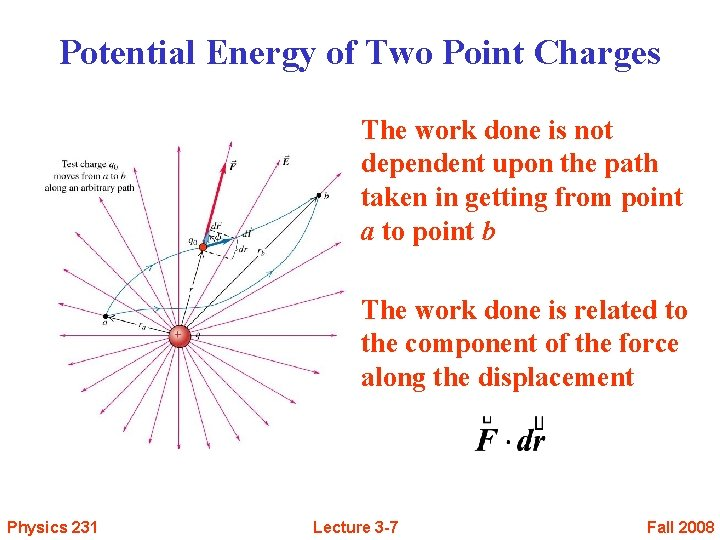Potential Energy of Two Point Charges The work done is not dependent upon the path taken in getting from point a to point b The work done is related to the component of the force along the displacement Physics 231 Lecture 3 -7 Fall 2008Potential Energy Looking at the work done we notice that there is the same functional at points a and b and that we are taking the difference We define this functional to be the potential energy The signs of the charges are included in the calculation The potential energy is taken to be zero when the two charges are infinitely separated Physics 231 Lecture 3 -8 Fall 2008A System of Point Charges Suppose we have more than two charges Have to be careful of the question being asked Two possible questions: 1) Total Potential energy of one of the charges with respect to remaining charges or 2) Total Potential Energy of the System Physics 231 Lecture 3 -9 Fall 2008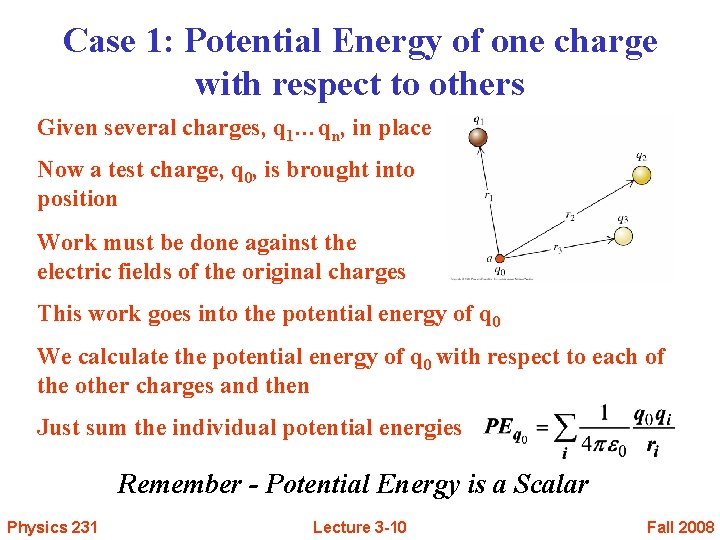Case 1: Potential Energy of one charge with respect to others Given several charges, q 1…qn, in place Now a test charge, q 0, is brought into position Work must be done against the electric fields of the original charges This work goes into the potential energy of q 0 We calculate the potential energy of q 0 with respect to each of the other charges and then Just sum the individual potential energies Remember - Potential Energy is a Scalar Physics 231 Lecture 3 -10 Fall 2008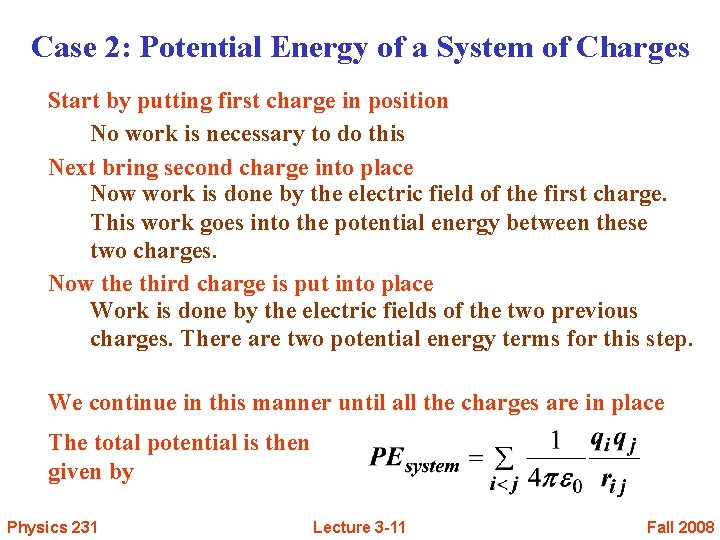Case 2: Potential Energy of a System of Charges Start by putting first charge in position No work is necessary to do this Next bring second charge into place Now work is done by the electric field of the first charge. This work goes into the potential energy between these two charges. Now the third charge is put into place Work is done by the electric fields of the two previous charges. There are two potential energy terms for this step. We continue in this manner until all the charges are in place The total potential is then given by Physics 231 Lecture 3 -11 Fall 2008Example 1 Two test charges are brought separately to the vicinity of a positive charge Q Charge +q is brought to pt A, a distance r from Q Q Charge +2 q is brought to pt B, a distance 2 r from Q Q r q A 2 r B 2 q I) Compare the potential energy of q (UA) to that of 2 q (UB) (b) UA = UB (c) UA > UB (a) UA < UB II) Suppose charge 2 q has mass m and is released from rest from the above position (a distance 2 r from Q). What is its velocity vf as it approaches r = ∞ ? (a) Physics 231 (b) (c) Lecture 3 -12 Fall 2008Example 2 Two test charges are brought separately to the vicinity of a positive charge Q Charge +q is brought to pt A, a distance r from Q Q Charge +2 q is brought to pt B, a distance 2 r from Q Q r q A 2 r B 2 q I) Compare the potential energy of q (UA) to that of 2 q (UB) (b) UA = UB (c) UA > UB (a) UA < UB The potential energy of q is proportional to Qq/r The potential energy of 2 q is proportional to Q(2 q)/(2 r) = Qq/r Therefore, the potential energies UA and UB are EQUAL!!! Physics 231 Lecture 3 -13 Fall 2008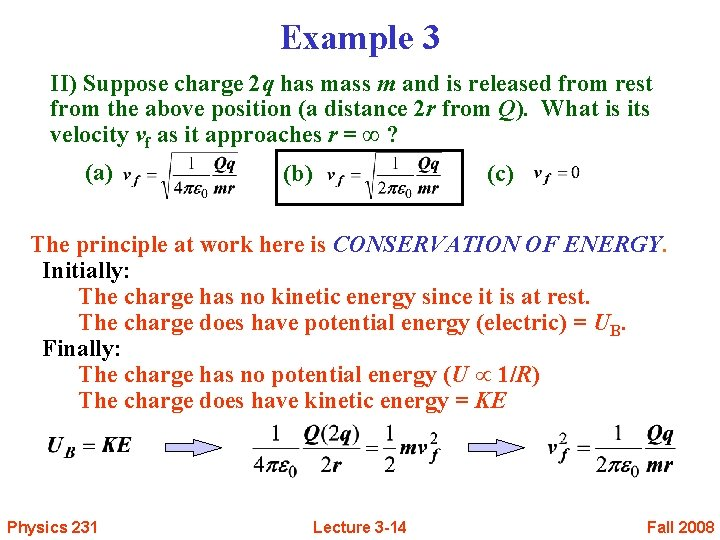Example 3 II) Suppose charge 2 q has mass m and is released from rest from the above position (a distance 2 r from Q). What is its velocity vf as it approaches r = ∞ ? (a) (b) (c) The principle at work here is CONSERVATION OF ENERGY. Initially: The charge has no kinetic energy since it is at rest. The charge does have potential energy (electric) = UB. Finally: The charge has no potential energy (U 1/R) The charge does have kinetic energy = KE Physics 231 Lecture 3 -14 Fall 2008Electric Potential Recall Case 1 from before The potential energy of the test charge, q 0, was given by Notice that there is a part of this equation that would remain the same regardless of the test charge, q 0, placed at point a The value of the test charge can be pulled out from the summation Physics 231 Lecture 3 -15 Fall 2008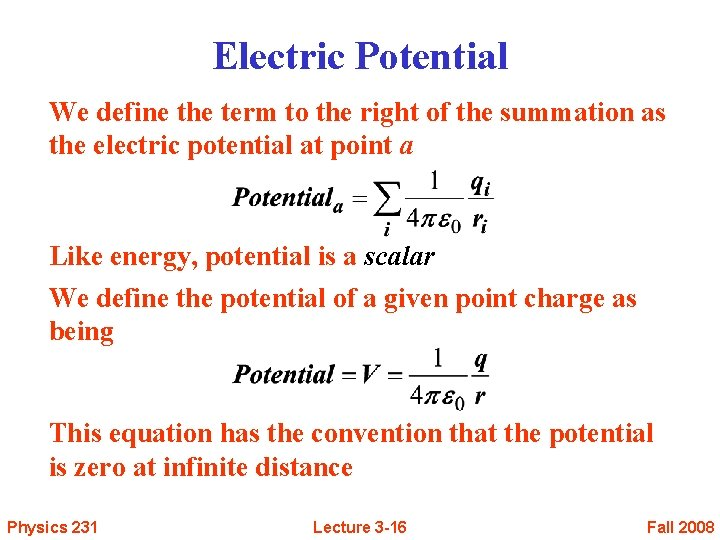Electric Potential We define the term to the right of the summation as the electric potential at point a Like energy, potential is a scalar We define the potential of a given point charge as being This equation has the convention that the potential is zero at infinite distance Physics 231 Lecture 3 -16 Fall 2008Electric Potential The potential at a given point Represents the potential energy that a positive unit charge would have, if it were placed at that point It has units of Physics 231 Lecture 3 -17 Fall 2008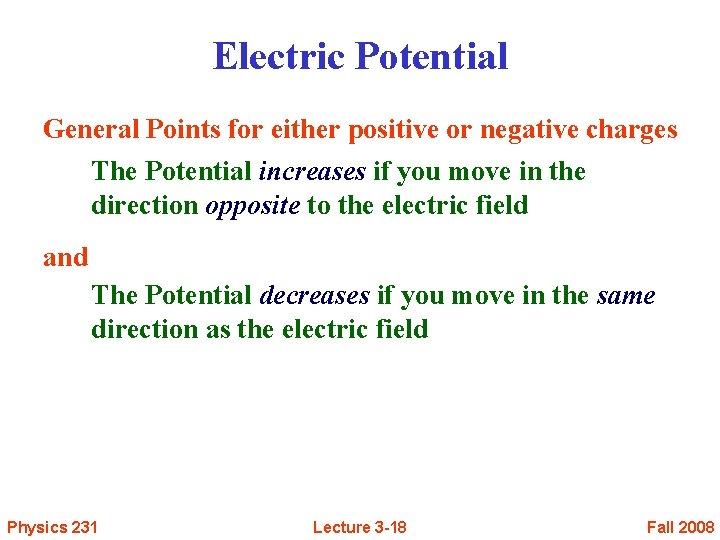Electric Potential General Points for either positive or negative charges The Potential increases if you move in the direction opposite to the electric field and The Potential decreases if you move in the same direction as the electric field Physics 231 Lecture 3 -18 Fall 2008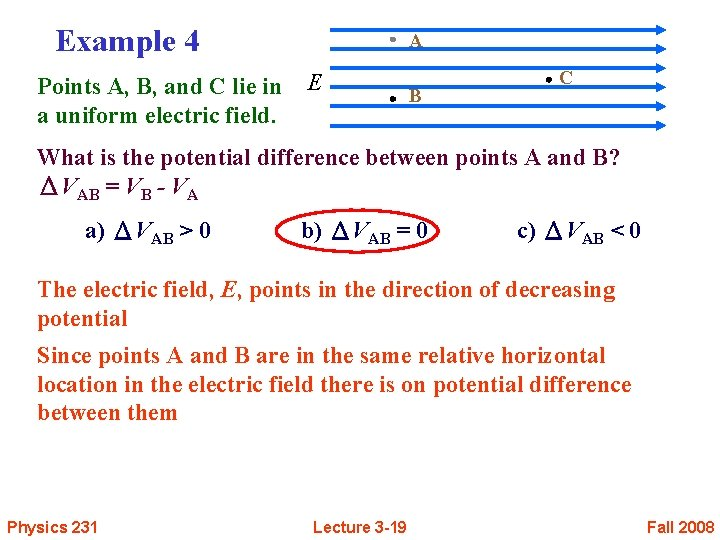Example 4 Points A, B, and C lie in a uniform electric field. A E B C What is the potential difference between points A and B? ΔVAB = VB - VA a) ΔVAB > 0 b) ΔVAB = 0 c) ΔVAB < 0 The electric field, E, points in the direction of decreasing potential Since points A and B are in the same relative horizontal location in the electric field there is on potential difference between them Physics 231 Lecture 3 -19 Fall 2008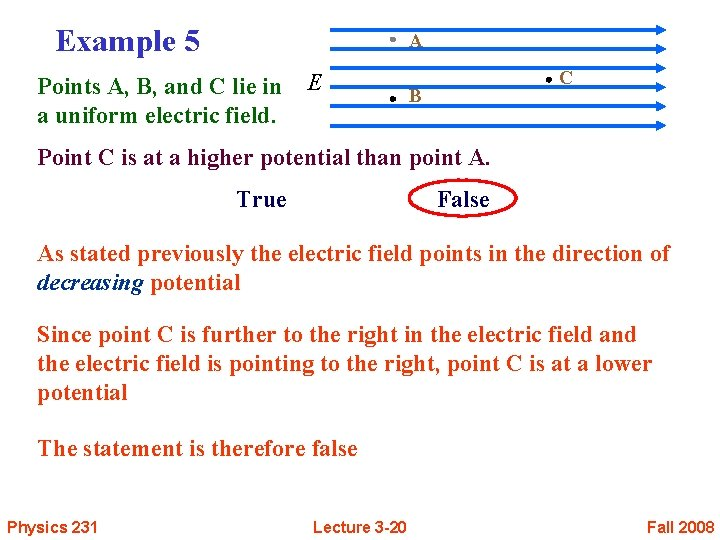Example 5 A Points A, B, and C lie in a uniform electric field. E C B Point C is at a higher potential than point A. True False As stated previously the electric field points in the direction of decreasing potential Since point C is further to the right in the electric field and the electric field is pointing to the right, point C is at a lower potential The statement is therefore false Physics 231 Lecture 3 -20 Fall 2008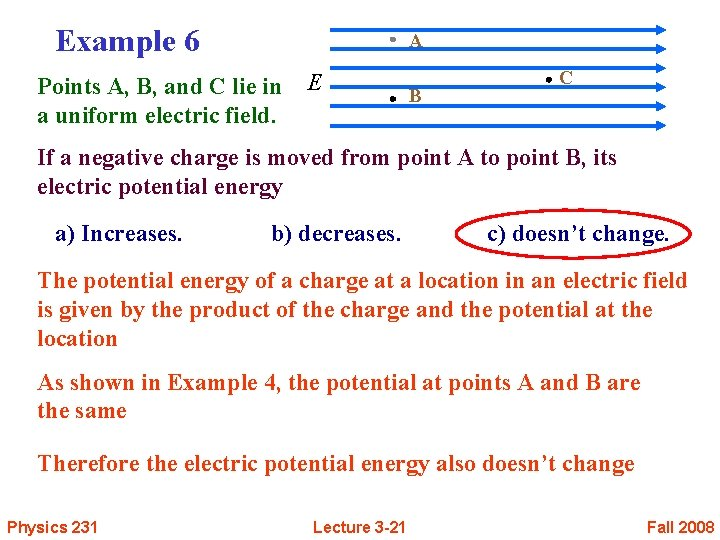Example 6 A Points A, B, and C lie in a uniform electric field. E B C If a negative charge is moved from point A to point B, its electric potential energy a) Increases. b) decreases. c) doesn’t change. The potential energy of a charge at a location in an electric field is given by the product of the charge and the potential at the location As shown in Example 4, the potential at points A and B are the same Therefore the electric potential energy also doesn’t change Physics 231 Lecture 3 -21 Fall 2008Example 7 Points A, B, and C lie in a uniform electric field. A E B C Compare the potential differences between points A and C and points B and C. a) VAC > VBC b) VAC = VBC c) VAC < VBC In Example 4 we showed that the potential at points A and B were the same Therefore the potential difference between A and C and the potential difference between points B and C are the same Also remember that potential and potential energy are scalars and directions do not come into play Physics 231 Lecture 3 -22 Fall 2008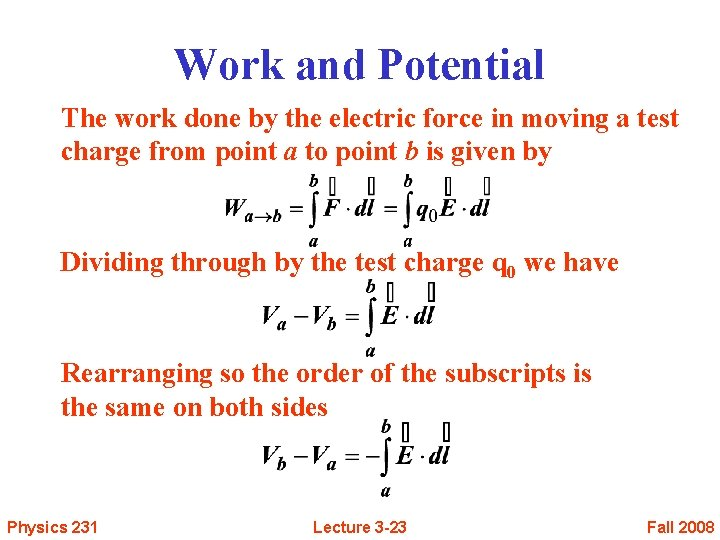Work and Potential The work done by the electric force in moving a test charge from point a to point b is given by Dividing through by the test charge q 0 we have Rearranging so the order of the subscripts is the same on both sides Physics 231 Lecture 3 -23 Fall 2008Potential From this last result We get We see that the electric field points in the direction of decreasing potential We are often more interested in potential differences as this relates directly to the work done in moving a charge from one point to another Physics 231 Lecture 3 -24 Fall 2008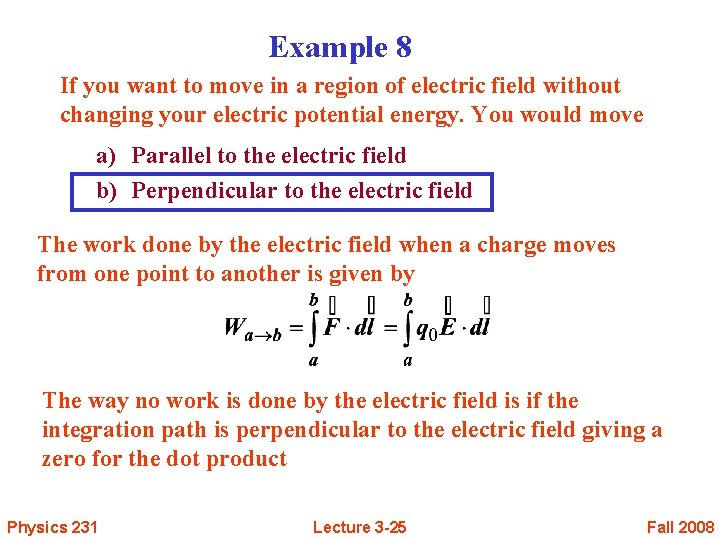Example 8 If you want to move in a region of electric field without changing your electric potential energy. You would move a) Parallel to the electric field b) Perpendicular to the electric field The work done by the electric field when a charge moves from one point to another is given by The way no work is done by the electric field is if the integration path is perpendicular to the electric field giving a zero for the dot product Physics 231 Lecture 3 -25 Fall 2008Example 9 A positive charge is released from rest in a region of electric field. The charge moves: a) towards a region of smaller electric potential b) along a path of constant electric potential c) towards a region of greater electric potential A positive charge placed in an electric field will experience a force given by But E is also given by Therefore Since q is positive, the force F points in the direction opposite to increasing potential or in the direction of decreasing potential Physics 231 Lecture 3 -26 Fall 2008Units for Energy There is an additional unit that is used for energy in addition to that of joules A particle having the charge of e (1. 6 x 10 -19 C) that is moved through a potential difference of 1 Volt has an increase in energy that is given by Physics 231 Lecture 3 -27 Fall 2008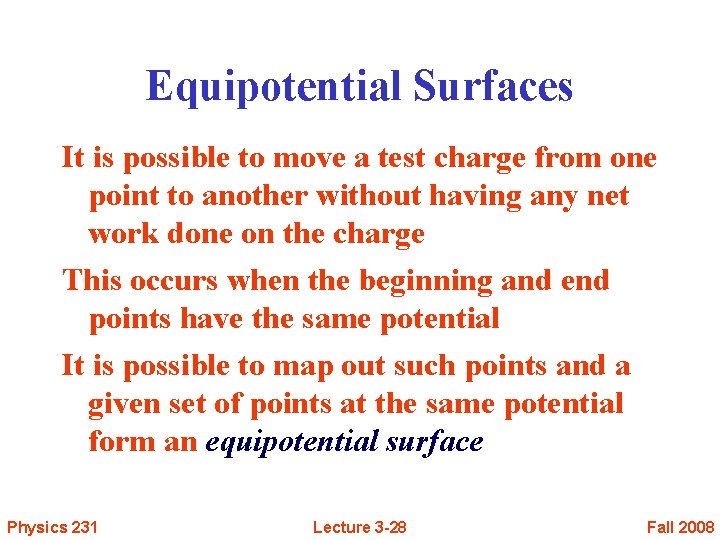Equipotential Surfaces It is possible to move a test charge from one point to another without having any net work done on the charge This occurs when the beginning and end points have the same potential It is possible to map out such points and a given set of points at the same potential form an equipotential surface Physics 231 Lecture 3 -28 Fall 2008Equipotential Surfaces Examples of equipotential surfaces Point Charge Physics 231 Two Positive Charges Lecture 3 -29 Fall 2008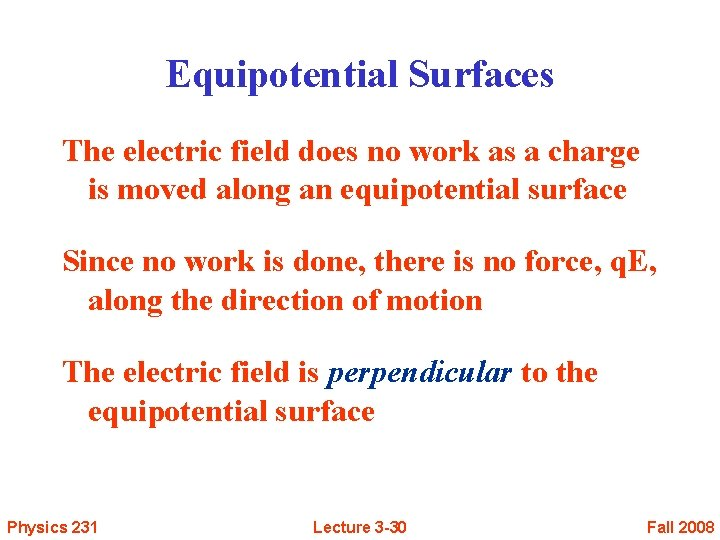Equipotential Surfaces The electric field does no work as a charge is moved along an equipotential surface Since no work is done, there is no force, q. E, along the direction of motion The electric field is perpendicular to the equipotential surface Physics 231 Lecture 3 -30 Fall 2008What about Conductors In a static situation, the surface of a conductor is an equipotential surface But what is the potential inside the conductor if there is a surface charge? We know that E = 0 inside the conductor This leads to Physics 231 Lecture 3 -31 Fall 2008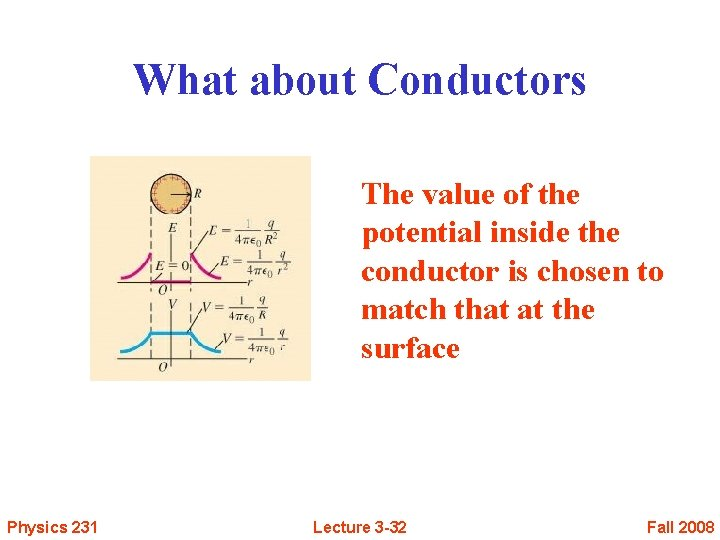What about Conductors The value of the potential inside the conductor is chosen to match that at the surface Physics 231 Lecture 3 -32 Fall 2008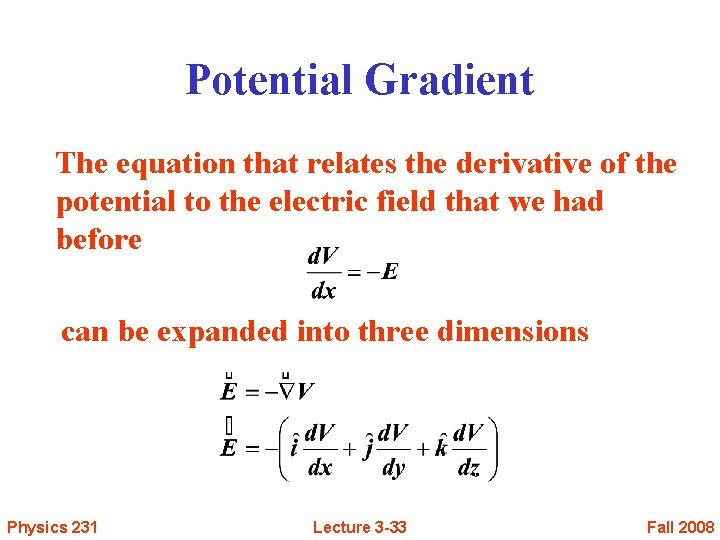Potential Gradient The equation that relates the derivative of the potential to the electric field that we had before can be expanded into three dimensions Physics 231 Lecture 3 -33 Fall 2008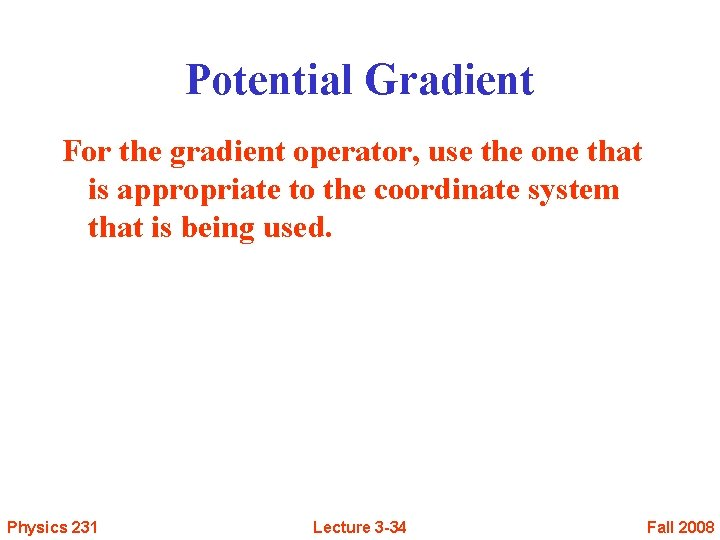Potential Gradient For the gradient operator, use the one that is appropriate to the coordinate system that is being used. Physics 231 Lecture 3 -34 Fall 2008Example 10 The electric potential in a region of space is given by The x-component of the electric field Ex at x = 2 is (a) Ex = 0 (b) Ex > 0 (c) Ex < 0 We know V(x) “everywhere” To obtain Ex “everywhere”, use Physics 231 Lecture 3 -35 Fall 2008